# Dividend Decision: Dividend Theories

Some of the major different theories of dividend in financial management are as follows: 1. Walter’s model

2. Gordon’s model

3. Modigliani and Miller’s hypothesis.

### 1. Walter’s model:

Professor James E. Walterargues that the choice of dividend policies almost always affects the value of the enterprise. His model shows clearly the importance of the relationship between the firm’s internal rate of return (r) and its cost of capital (k) in determining the dividend policy that will maximise the wealth of shareholders.

Walter’s model is based on the following assumptions:

1. The firm finances all investment through retained earnings; that is debt or new equity is not issued;
2. The firm’s internal rate of return (r), and its cost of capital (k) are constant;
3. All earnings are either distributed as dividend or reinvested internally immediately.
4. Beginning earnings and dividends never change. The values of the earnings pershare (E), and the divided per share (D) may be changed in the model to determine results, but any given values of E and D are assumed to remain constant forever in determining a given value.
5. The firm has a very long or infinite life.

Walter’s formula to determine the market price per share (P) is as follows:

P = D/K +r(E-D)/K/K

The above equation clearly reveals that the market price per share is the sum of the present value of two sources of income:

i) The present value of an infinite stream of constant dividends, (D/K) and

ii) The present value of the infinite stream of stream gains.

[r (E-D)/K/K]

#### Criticism:

Walter’s model is quite useful to show the effects of dividend policy on an all equity firm under different assumptions about the rate of return. However, the simplified nature of the model can lead to conclusions which are net true in general, though true for Walter’s model.

The criticisms on the model are as follows:

1. Walter’s model of share valuation mixes dividend policy with investment policy of the firm. The model assumes that the investment opportunities of the firm are financed by retained earnings only and no external financing debt or equity is used for the purpose when such a situation exists either the firm’s investment or its dividend policy or both will be sub-optimum. The wealth of the owners will maximise only when this optimum investment in made.
2. Walter’s model is based on the assumption that r is constant. In fact decreases as more investment occurs. This reflects the assumption that the most profitable investments are made first and then the poorer investments are made.

The firm should step at a point where r = k. This is clearly an erroneous policy and fall to optimise the wealth of the owners.

1. A firm’s cost of capital or discount rate, K, does not remain constant; it changes directly with the firm’s risk. Thus, the present value of the firm’s income moves inversely with the cost of capital. By assuming that the discount rate, K is constant, Walter’s model abstracts from the effect of risk on the value of the firm.

### 2. Gordon’s Model:

One very popular model explicitly relating the market value of the firm to dividend policy is developed by Myron Gordon.

#### Assumptions:

Gordon’s model is based on the following assumptions.

1. The firm is an all Equity firm
2. No external financing is available
3. The internal rate of return (r) of the firm is constant.
4. The appropriate discount rate (K) of the firm remains constant.
5. The firm and its stream of earnings are perpetual
6. The corporate taxes do not exist.
7. The retention ratio (b), once decided upon, is constant. Thus, the growth rate (g) = br is constant forever.
8. K > br = g if this condition is not fulfilled, we cannot get a meaningful value for the share.

According to Gordon’s dividend capitalisation model, the market value of a share (Pq) is equal to the present value of an infinite stream of dividends to be received by the share. Thus: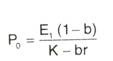The above equation explicitly shows the relationship of current earnings (E,), dividend policy, (b), internal profitability (r) and the all-equity firm’s cost of capital (k), in the determination of the value of the share (P0).

### 3. Modigliani and Miller’s hypothesis:

According to Modigliani and Miller (M-M), dividend policy of a firm is irrelevant as it does not affect the wealth of the shareholders. They argue that the value of the firm depends on the firm’s earnings which result from its investment policy.

Thus, when investment decision of the firm is given, dividend decision the split of earnings between dividends and retained earnings is of no significance in determining the value of the firm. M – M’s hypothesis of irrelevance is based on the following assumptions.

1. The firm operates in perfect capital market
2. Taxes do not exist
3. The firm has a fixed investment policy
4. Risk of uncertainty does not exist. That is, investors are able to forecast future prices and dividends with certainty and one discount rate is appropriate for all securities and all time periods. Thus, r = K = Ktfor all t.

Under M – M assumptions, r will be equal to the discount rate and identical for all shares. As a result, the price of each share must adjust so that the rate of return, which is composed of the rate of dividends and capital gains, on every share will be equal to the discount rate and be identical for all shares.

Thus, the rate of return for a share held for one year may be calculated as follows: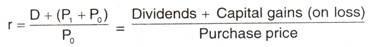Where P^ is the market or purchase price per share at time 0, P, is the market price per share at time 1 and D is dividend per share at time 1. As hypothesised by M – M, r should be equal for all shares. If it is not so, the low-return yielding shares will be sold by investors who will purchase the high-return yielding shares.

This process will tend to reduce the price of the low-return shares and to increase the prices of the high-return shares. This switching will continue until the differentials in rates of return are eliminated. This discount rate will also be equal for all firms under the M-M assumption since there are no risk differences.

From the above M-M fundamental principle we can derive their valuation model as follows: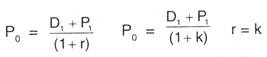Multiplying both sides of equation by the number of shares outstanding (n), we obtain the value of the firm if no new financing exists.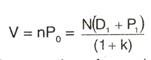If the firm sells m number of new shares at time 1 at a price of P^, the value of the firm at time 0 will be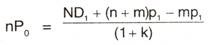The above equation of M – M valuation allows for the issuance of new shares, unlike Walter’s and Gordon’s models. Consequently, a firm can pay dividends and raise funds to undertake the optimum investment policy. Thus, dividend and investment policies are not confounded in M – M model, like waiter’s and Gordon’s models.

#### Criticism:

Because of the unrealistic nature of the assumption, M-M’s hypothesis lacks practical relevance in the real world situation. Thus, it is being criticised on the following grounds.

1. The assumption that taxes do not exist is far from reality.
2. M-M argue that the internal and external financing are equivalent. This cannot be true if the costs of floating new issues exist.
3. According to M-M’s hypothesis the wealth of a shareholder will be same whether the firm pays dividends or not. But, because of the transactions costs and inconvenience associated with the sale of shares to realise capital gains, shareholders prefer dividends to capital gains.
4. Even under the condition of certainty it is not correct to assume that the discount rate (k) should be same whether firm uses the external or internal financing.

If investors have desire to diversify their port folios, the discount rate for external and internal financing will be different.

1. M-M argues that, even if the assumption of perfect certainty is dropped and uncertainty is considered, dividend policy continues to be irrelevant. But according to number of writers, dividends are relevant under conditions of uncertainty.

## One thought on “Dividend Decision: Dividend Theories”

error: Content is protected !!